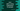# How to split a string in Dart### Introduction :

Splitting a string is available in almost all programming languages. Splitting divides a string in different parts based on one expression or rule. In dart also, we have an inbuilt splitting method known as split(). We can define it as below :

``````List split(Pattern pattern);
``````

It takes one Pattern as input. Based on this pattern, it splits the string into several small substrings. Then it put them back in a List and returns it. In this tutorial, we will learn how to use Split in Dart with different examples. Let’s take a look :

### Basic example of split :

Let’s start with a simple example of how to use split in Dart. We will split the string ‘The quick brown fox jumps over the lazy dog’ into words.

``````main(List args) {
var input = "The quick brown fox jumps over the lazy dog";

print(input.split(" "));
}
``````

It will print the below output :

``````[The, quick, brown, fox, jumps, over, the, lazy, dog]
``````

### Explanation :

In this example, we have passed one blank space(” ”) as the parameter to the split() method. So, it divided all words to a list of words using the blank space as separator. Instead of using a space, if we use empty space (""), what result it will print out?

``````main(List args) {
var input = "The quick brown fox jumps over the lazy dog";

print(input.split(""));
}
``````

Output :

``````[T, h, e,  , q, u, i, c, k,  , b, r, o, w, n,  , f, o, x,  , j, u, m, p, s,  , o, v, e, r,  , t, h, e,  , l, a, z, y,  , d, o, g]
``````

As you can see, it split the string into the letters. One thing to note is that blank spaces are also included in the split list.

### Using a regular expression :

We can pass any simple or complex Regular expression as a parameter to split() method. It will do the splitting based on the regular expression. Let me show you with an example :

``````main(List args) {
var input = "The1quick5brown6fox0jumps7over8the2lazy4dog";

print(input.split(new RegExp(r"[0-9]")));
}
``````

It will print the below output :

``````[The, quick, brown, fox, jumps, over, the, lazy, dog]
``````

As you can see the words are extracted out from the string. Because r”[0-9]” means any number from 0 to 9 and thus the splitting is done for any one digit number that is found in the string.

## Conclusion :

We have learnt different ways to split a string in Dart. Try to run these examples and if you have any queries or anything you want to add, drop a comment below.